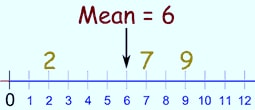Alert: You are not allowed to copy content or view source
IMO Result Declared Check here | IMO Level 2 Exam Timing & Guidelines Check here | Register for Maths, Science, English, GK Olympiad Exam Click here | Check Olympiad Exam Dates here | Buy Practice Papers for IMO, IOM, HEO, IOEL etc here | Login here to participate in all India free mock test on every Saturday

# AverageA calculated "central" value of a set of numbers. To calculate: add up all the numbers, then divide by how many numbers there are. Example: what is the average of 2, 7 and 9? Add the numbers: 2 + 7 + 9 = 18 Divide by how many numbers (i.e. we added 3 nu

70%